# Normal force

View a complete list of force types

## Definition

QUICK PHRASES: force preventing objects from going into each other, measure of how pressed together two bodies are, component of contact force orthogonal to surface of contact, component of contact force perpendicular to contact surface, what's commonly mistaken for weight

### Intensional definition

Normal force, sometimes called normal reaction, is the component of contact force between two bodies acting in a direction perpendicular to the plane of contact (or the tangent plane at the point of contact). It is in the outward direction on both bodies. The magnitudes of the normal force on both bodies are equal and the directions are opposite, in keeping with Newton's third law.

### Tendency-based definition

Normal force is the force exerted by two bodies on each other at a surface of contact that is intended to precisely counteract any tendency the bodies have of moving into each other.

## Situational examples

Example Direction of force on each body Magnitude of force Reason Picture
A block placed on a fixed horizontal surface. Upward on the block, downward on the surface Equal to the weight of the block, which is the product of the mass and acceleration due to gravity The net vertical force on the block should be zero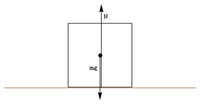A block placed on a fixed inclined plane with angle$\theta$ with the horizontal: see sliding motion along a frictionless inclined plane and sliding motion along an inclined plane Upward/outward on the block, downward/inward on the surface The product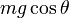$mg \cos \theta$, where$m$ is the mass of the block,$g$ is the acceleration due to gravity The normal force must balance the component of gravitational force that is trying to send the block into the inclined plane.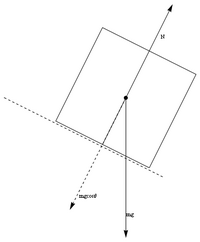A person lifting a book placed on his horizontal palm Upward on the book, downward on the palm$m(g + a)$ where$g$ is the acceleration due to gravity and$a$ is the upward acceleration. If the person is lifting the book with constant speed, it is just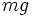$mg$. If the speed is reducing, the normal force is less than$mg$. Note that the normal force does not cancel$mg$ but rather is determined so as to create the necessary acceleration.
Two blocks sliding down, adjacent to each other on an inclined plane. Assume that they do not get separated. The block farther down exerts a normal force up the inclined plane on the other block, and the block further up exerts a downward force on the lower blocks. Fill this in later. Note that the normal force between the blocks is not set to ensure zero acceleration (hence, it does not balance out external forces in that direction), but it is set to ensure that the blocks accelerate equally, so that relative acceleration is still zero. Obtained by solving system of equationsA block on a circular incline, sliding down along the incline: see sliding motion along a frictionless circular incline and sliding motion along a circular incline Upward/outward on the block, downward/inward on the incline surface$mg \cos \theta - (mv^2/r)$, where$mg\cos \theta$ is the component of gravitational force along the normal direction, and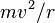$mv^2/r$ is the net centripetal force needed for the block to continue to stay on the circular incline. Newton's second law. Note that here,$N$ does not balance external forces in the normal direction, because we want the net force in the normal direction to be, not zero, but the centripetal force.

## Confusion with Newton's third law

The normal force between two bodies exerted through a surface of contact depends on the other forces acting on these bodies that are creating a tendency for the bodies to move into each other. In fact, it adjusts itself in magnitude to precisely counteract the external forces along the normal direction. Hence, it is equal and opposite to these external forces and is sometimes called a reaction force.

However, the normal force is not a reaction to the external force in the sense of Newton's third law, even though it is (in many typical situations) equal and opposite to the external force.

Rather, the normal forces that the two bodies exert on each other form an action-reaction pair. Here is an elaboration using the previous examples:

Example False interpretation of Newton's third law Correct interpretation of Newton's third law
A block placed on a fixed horizontal surface The upward normal force exerted by the surface on the block forms an action-reaction pair with the downward gravitational force on the block The upward normal force exerted by the surface on the block, and the downward normal force exerted by the block on the surface, form an action-reaction pair. The gravitational force exerted by the earth on the block and the gravitational force exerted by the block on the earth form another action-reaction pair.
A block placed on a fixed inclined plane with angle$\theta$ with the horizontal The upward/outward normal force exerted by the surface on the block forms an action-reaction pair with the component of gravitational force exerted on the block in the inward direction into the plane. The upward/outward normal force exerted by the surface on the block forms an action-reaction pair with an inward/downward normal force exerted by the block on the surface.
KEY POINT TO REMEMBER: Two different forces acting on the same body cannot form an action-reaction pair. The two forces of an action-reaction pair must act on different bodies.

## Units and dimensions

Normal force is a form of force and hence has the same dimensions and units as all forces do. The MLT dimensions are$MLT^{-2}$ and the SI unit is$N$ (Newton) or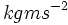$kgms^{-2}$. Another unit typically used is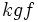$kgf$, which is the weight of$1kg$ of material. Other units used include units obtained by replacing$kg$ by other measures of weight,$m$ by other measures of length, and$s$ by other measures of time.

## Related forces

• Friction: It is the component of contact force along the plane of contact.
• Tension: Tension is a force exerted by a taut string on an object at its end, pulling the object inward.
• Spring force: Spring force is a force exerted by a spring on an object attached at its end. When the spring is extended, the force is inward, analogous to tension, while when the spring is compressed, this acts outward, analogous to normal force.
• Pressure: This is the normal force per unit area, or the differential of the normal force with respect to area. The normal force exerted through a surface of contact is the integral of the pressure with respect to area over the entire surface of contact.
• Stress: This is force per unit area that tends to have a deforming effect, and the force could be arising from a normal force.

## Subtleties

### Distribution over a flat surface of contact

The normal force acting through a surface of contact is the sum of forces acting on subparts of the surface. The magnitude of normal force per unit area is termed pressure. If we denote the pressure at a point on the surface of contact by$P$, then the total normal force can be given by integrating this pressure over the entire area of contact: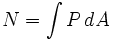$N = \int P \, dA$

where$dA$ is the area element being integrated over. If the pressure is uniform through the surface of contact, then we have: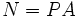$N = PA$

In general, the pressure need not be uniform over the entire surface of contact.

### Torque due to normal force

Further information: Torque due to normal force

Since the normal force is distributed over the entire surface of contact, different parts of it make different contributions to the torque. To determine the overall torque, we need to integrate, over the area of contact, the torque generated per unit area of contact.

In many cases of interest, the torque due to normal force is zero. This happens when the normal force is uniformly distributed over the surface and the surfaces is symmetric about the normal line to the surface through the center of mass. For many of these situations, a simplification is made: the normal force is shown as a force with a single line of action, namely, the normal line through the center of mass, and this is used to conclude that the normal force is zero.

### Normal force for a non-flat surface of contact

Consider a ball resting on/in a hemispherical surface of the same shape (so that there is a good fit). In this case, the surface of contact is not flat and there are different normal directions to different parts of the surface. The net direction of normal force in such situations is unclear. The normal force can be determined by the same integration as for a flat surface: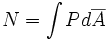$N =\int P d\overline{A}$

except that now the area element is treated as a vector pointing in the normal direction at the point of contact, and the integration is thus a vector integration.

### Normal force for a hinge/point of contact

In some cases, the surface of contact is very small and, in the geometric approximation, is a point or a line rather than a surface. (In reality, it is still a surface, albeit one of very small area). The concept of normal force still makes sense, and it acts in the direction perpendicular to the tangent plane through the point of contact.

In case of irregular surfaces, however,there may not be a well-defined tangent plane, and in this case, there may be some indeterminacy in the direction of normal force. The actual direction of normal force depends on the normal direction to the surface area as it is actually formed due to microscopic deformations. This becomes of significance in situations such as rolling friction and some examples of banking.

### Normal impulse

In head-on collisions, there is a huge normal force between the colliding bodies for a short interval of time. For such situations, it is not customary to calculate the value of the normal force over this short time interval, but the normal impulse, which is the integral of this normal force over the time duration of the collision, is important.

In oblique collisions, there is both a normal impulse and a frictional impulse, which is the impulse due to frictional force. The frictional impulse is the coefficient of kinetic friction times the normal impulse.

## Work and energy

Normal force can do work if the normal force causes motion, e.g., with one body pushing the other body in some direction. It does not do work if the normal force is balanced by other forces perpendicular to the plane of contact that result in no net motion in that direction.

For instance, if a person lifts a book by placing it on the palm of her hand and moving her palm upward, then the normal force between the palm and the book causes the book to be lifted. The overall work done by the normal force is obtained by using the expression$\int F \cdot ds$ where$F$ is the normal force. Part of this work is converted to potential energy, since it combats the gravitational force acting on the book, namely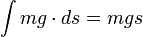$\int mg \cdot ds = mgs$ where$s$ is the total height gained by the book. The rest of the work is converted into kinetic energy, which is the energy that the book acquires because of its speed. This is given by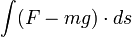$\int (F - mg) \cdot ds$.

If a person pushes a block forward on a frictionless floor, the work done by the normal force is$\int F \cdot ds$, all of which is converted into the block's kinetic energy. If, however, the person pushes the block forward on a floor with friction, part of the work done, namely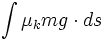$\int \mu_k mg \cdot ds$, is dissipated in kinetic friction, and the remaining is stored in the form of the block's kinetic energy.